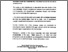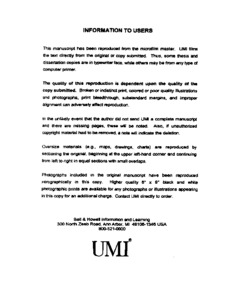Title:

# Nonparametric prediction in survey sampling and its application to the nonresponse problem

Crisalli, Anthony N (1999) Nonparametric prediction in survey sampling and its application to the nonresponse problem. PhD thesis, Concordia University.Preview
Text (application/pdf)
NQ47726.pdf
4MB

## Abstract

Nonparametric regression provides an important tool towards exploring the relationship between a dependent variable and the independent variable(s) without assuming a functional form between the variables. This thesis incorporates the nonparametric regression methodology in the context of estimation of a finite population mean and/or total. The resulting estimator is called the generalized smoothing (GSE) in contrast to the, model based generalized regression estimator (GRE), developed by Särndal (1972). The theory available for GRE is extended for the GSE. We replace model-based predictors by those based on nonparametric regression. The first objective is to investigate the merits of GSE over GRE in the simple random samples with respect to different criteria. It is shown that GSE is design-consistent and asymptotically design-unbiased. Furthermore, Monte Carlo simulations are carried out to, compare the GSE and the GRE with respect to several criteria, such as the Bias and the Mean Square Error (MSE). The second objective is to extend the above methodology in situations of non-response. In this case a probability response model for the respondents is assumed; the case of each sample unit not responding with equal probability is considered as a particular case. Monte Carlo simulations of the proposed procedures are presented so as to understand the behavior the GSE, and the probability response model. The linear regression model is ubiquitous in the sample survey literature, an assumption that is untenable in practice. In this dissertation we illustrate that it is feasible to estimate a finite population mean (also in the presence of nonresponse) with a nonparametric regression model. The simulations demonstrate in both the cases of full response and nonresponse, that if the underlying point scatter is linear then the GRE is the best choice. On the other hand if the point scatter is not linear, a situation often met in practice, the GSE seems to outperform the ORE, in terms of the benchmark criteria of MSE and bias.

Divisions: Concordia University > School of Graduate Studies Thesis (PhD) Crisalli, Anthony N x, 159 leaves ; 29 cm. Concordia University Ph. D. School of Graduate Studies 1999 Chaubey, Yogendra P QA 276.6 C75 1999 961 Concordia University Library 27 Aug 2009 17:15 13 Jul 2020 19:48 https://concordiauniversity.on.worldcat....
All items in Spectrum are protected by copyright, with all rights reserved. The use of items is governed by Spectrum's terms of access.

Repository Staff Only: item control pageResearch related to the current document (at the CORE website)
Back to top